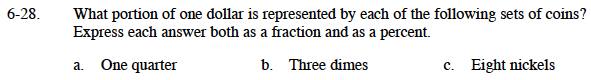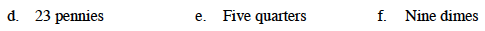### Home > MC1 > Chapter 6 > Lesson 6.1.3 > Problem6-28

6-28.Remember that one dollar is 100 cents and one quarter is 25 cents.

$25\%,\;\frac{25}{100}\;=\;\frac{1}{4}$

How many cents is three dimes?
How can you write this as a portion of 100?

30%.
How do you write 30% as a fraction?

Eight nickels is equal to 40 cents.
Use parts (a) and (b) to help you express this as a fraction and a percent.If one quarter (25 cents) is 25% of a dollar, what percent is 23 cents?

By how much do you multiply one quarter to get five quarters?
Since you dealt with one quarter in part (a), you can use that as a guide for representing 5 quarters as a fraction and a percent.